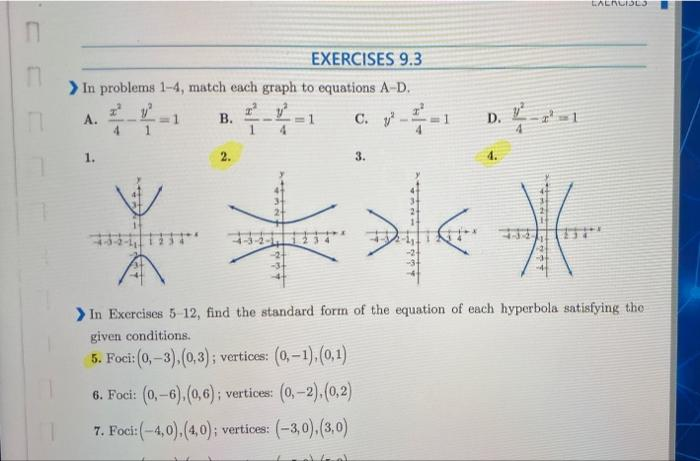# Question can anyone solve this three qestions plz ? LALALISLS m EXERCISES 9.3 > In problems 1-4, match each graph to equations A-D. A. 목 B. C. V VP D. 1. 2. 3. *** In Exercises 5-12, find the standard form of the equation of each hyperbola satisfying the given conditions. 5. Foci:(0, -3),(0,3); vertices: (0, -1),(0,1) 6. Foci: (0,–6),(0,6); vertices: (0,-2),(0,2) 7. Foci:(-4,0).(4,0); vertices: (-3,0),(3,0)